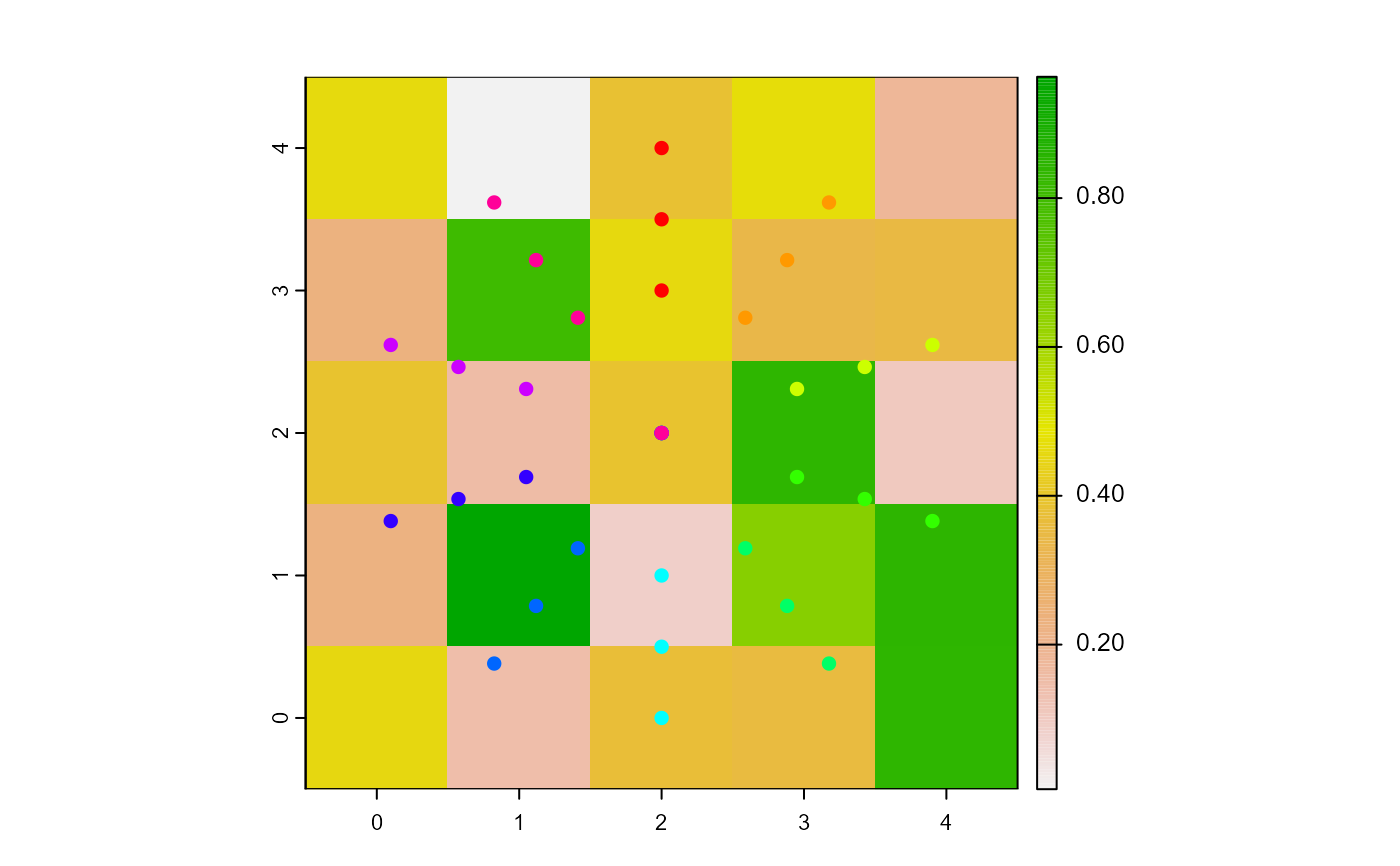Move the turtles backward of their headings' directions.

bk(turtles, dist, world, torus = FALSE, out = TRUE)

# S4 method for agentMatrix,numeric
bk(turtles, dist, world, torus = FALSE, out = TRUE)

## Arguments

turtles

AgentMatrix object representing the moving agents.

dist

Numeric. Vector of distances to move. Must be of length 1 or of length turtles.

world

WorldMatrix or worldArray object.

torus

Logical to determine if the world is wrapped. Default is torus = FALSE.

out

Logical. Determine if a turtle should move when torus = FALSE and its ending position will be outside of the world's extent. Default is out = TRUE.

## Value

AgentMatrix representing the turtles with updated coordinates and updated data for their previous coordinates prevX

and prevY.

## Details

If torus = FALSE and out = TRUE, world does not need to be provided.

     If a distance to move leads a turtle outside of the world's extent

and torus = TRUE, the turtle is

relocated on the other side of the world, inside its extent; if

torus = FALSE and out = TRUE, the turtle moves past the

world's extent; if torus = FALSE and out = FALSE, the

turtle does not move at all. In the event that a turtle does not move,

its previous coordinates are still updated with its position before

running bk() (i.e., its current position).

If a given dist value is negative, then the turtle moves

forward.

The turtles' headings are not affected by the function (i.e., the

turtles do not face backward).

https://ccl.northwestern.edu/netlogo/docs/dictionary.html#back

     <https://ccl.northwestern.edu/netlogo/docs/dictionary.html#jump>

Sarah Bauduin

## Examples

w1 <- createWorld(
minPxcor = 0, maxPxcor = 4, minPycor = 0, maxPycor = 4,
data = runif(25)
)
t1 <- createOTurtles(n = 10, world = w1)
plot(w1)
points(t1, col = of(agents = t1, var = "color"), pch = 16)

t1 <- fd(turtles = t1, dist = 2)
points(t1, col = of(agents = t1, var = "color"), pch = 16)
t1 <- bk(turtles = t1, dist = 1)
points(t1, col = of(agents = t1, var = "color"), pch = 16)
t1 <- fd(turtles = t1, dist = 0.5)
points(t1, col = of(agents = t1, var = "color"), pch = 16)